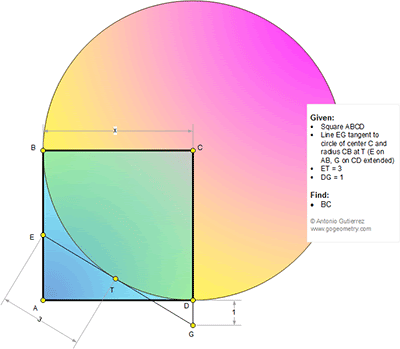## Friday, October 23, 2015

### Geometry Problem 1156: Square, Tangent Line, 90 Degrees, Metric Relations

Level: Mathematics Education, High School, Honors Geometry, College.

Click the diagram below for more details.1.Let u= ∠(BCE) and t= tan(u)
We have ET=x.tan(u)=3 ..... (1)
DG= x/cos(90-2.u)-x= x/sin(2u)-x
And ET/DG=3= tan(u)/(1/sin(2u)-1)
Replace sin(2u)=2t/(t^2+1) in above
We get 2.t^2/(t-1)^2=3 so t= √ (6)/( √ (6)+2)
Replace value of t in (1) we get x= 3+√ (6)

2.Let the intersection of EG & AD be called F and let FT=FD=y. From similar triangles FEA & FDG we can say that (x-3)/1=(x-y)/y and right triangle FEA gives us, (3+y)²=(x-3)²+(x-y)². Solving these two equations, we obtain the only admissible value of x as (3 +√6)

3.Purely Geometry solution

Let TG = p

Then from Pythagoras p^2 + x^2 = (x+1)^2

So p^2 = 2x+1. (1)

Now write the area of Tr. CEG in 2 ways

1/2 x(p+3) = 1/2 x (x+1)

So p = x - 2. and from (1)

(X-2)^2 = 2x + 1 so x^2 -6x + 3 = 0

x = 3 + or - sqrt 6

Sumith Peiris
Moratuwa
Sri Lanka

4.Alternatively since 2 altitudes of the Tr. ECG are equal the corresponding sides can easily be proved to be equal

Hence x+1 = p+3 and so on

5.Note that x=3+√ (6) is only acceptable solution of this problem.
Peter Tran

6.EC bisects angle BET (BEC)
So angles GCE = CEB = CEG
Thus GE = GC = 1 + x,
GT = GE - 3 = x - 2
Let GC be extended to meet circle at D'
So GT^2 = GD. GD' = 1.(1 + 2x) = 1 + 2x.
Follows (x- 2)^2 = 2x + 1, x^2 - 6x + 3 = 0 etc

7.I use the point F like the user Ajit did. My solution here:
8.